## Document Similarity in Machine Learning Text Analysis with ELMo

In this post we will look at using ELMo for computing similarity between text documents. Elmo is one of the word embeddings techniques that are widely used now. In the previous post we used TF-IDF for calculating text documents similarity. TF-IDF is based on word frequency counting. Both techniques can be used for converting text to numbers in information retrieval machine learning algorithms.The good tutorial that explains how ElMo is working and how it is built is Deep Contextualized Word Representations with ELMo
Another resource is at ELMo

We will however focus on the practical side of computing similarity between text documents with ELMo. Below is the code to accomplish this task. To compute elmo embeddings I used function from Analytics Vidhya machine learning post at learn-to-use-elmo-to-extract-features-from-text/

We will use cosine_similarity module from sklearn to calculate similarity between numeric vectors. It computes cosine similarity between samples in X and Y as the normalized dot product of X and Y.

```# -*- coding: utf-8 -*-

from sklearn.metrics.pairwise import cosine_similarity

import tensorflow_hub as hub
import tensorflow as tf

elmo = hub.Module("https://tfhub.dev/google/elmo/2", trainable=True)

def elmo_vectors(x):

embeddings=elmo(x, signature="default", as_dict=True)["elmo"]

with tf.Session() as sess:
sess.run(tf.global_variables_initializer())
sess.run(tf.tables_initializer())
# return average of ELMo features
return sess.run(tf.reduce_mean(embeddings,1))
```

Our data input will be the same as in previous post for TF-IDF: collection the sentences as an array. So each document here is represented just by one sentence.

```corpus=["I'd like an apple juice",
"An apple a day keeps the doctor away",
"Eat apple every day",
"We buy apples every week",
"We use machine learning for text classification",
"Text classification is subfield of machine learning"]

```

Below we do elmo embedding for each document and create matrix for all collection. If we print elmo_embeddings for i=0 we will get word embeddings vector [ 0.02739557 -0.1004054 0.12195794 … -0.06023929 0.19663551 0.3809018 ] which is numeric representation of the first document.

```elmo_embeddings=[]
print (len(corpus))
for i in range(len(corpus)):
print (corpus[i])
elmo_embeddings.append(elmo_vectors([corpus[i]]))

```

Finally we can print embeddings and similarity matrix

```print ( elmo_embeddings)
print(cosine_similarity(elmo_embeddings, elmo_embeddings))

[array([ 0.02739557, -0.1004054 ,  0.12195794, ..., -0.06023929,
0.19663551,  0.3809018 ], dtype=float32), array([ 0.08833811, -0.21392687, -0.0938901 , ..., -0.04924499,
0.08270906,  0.25595033], dtype=float32), array([ 0.45237526, -0.00928468,  0.5245862 , ...,  0.00988374,
-0.03330074,  0.25460464], dtype=float32), array([-0.14745474, -0.25623208,  0.20231596, ..., -0.11443609,
-0.03759   ,  0.18829307], dtype=float32), array([-0.44559947, -0.1429281 , -0.32497618, ...,  0.01917108,
-0.29726124, -0.02022664], dtype=float32), array([-0.2502797 ,  0.09800234, -0.1026585 , ..., -0.22239089,
0.2981896 ,  0.00978719], dtype=float32)]

The similarity matrix computed as :
[[0.9999998  0.609864   0.574287   0.53863835 0.39638174 0.35737067]
[0.609864   0.99999976 0.6036072  0.5824003  0.39648792 0.39825168]
[0.574287   0.6036072  0.9999998  0.7760986  0.3858403  0.33461633]
[0.53863835 0.5824003  0.7760986  0.9999995  0.4922789  0.35490626]
[0.39638174 0.39648792 0.3858403  0.4922789  0.99999976 0.73076516]
[0.35737067 0.39825168 0.33461633 0.35490626 0.73076516 1.0000002 ]]
```

Now we can compare this similarity matrix with matrix obtained with TF-IDF in prev post. Obviously they are different.

Thus, we calculated similarity between textual documents using ELMo. This post and previous post about using TF-IDF for the same task are great machine learning exercises. Because we use text conversion to numbers, document similarity in many algorithms of information retrieval, data science or machine learning.

## Text Clustering with doc2vec Word Embedding Machine Learning Model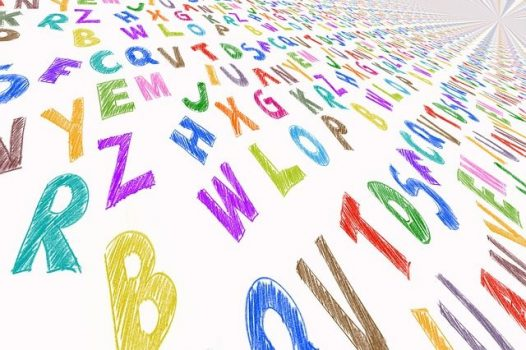In this post we will look at doc2vec word embedding model, how to build it or use pretrained embedding file. For practical example we will explore how to do text clustering with doc2vec model.

## Doc2vec

Doc2vec is an unsupervised computer algorithm to generate vectors for sentence/paragraphs/documents. The algorithm is an adaptation of word2vec which can generate vectors for words. Below you can see frameworks for learning word vector word2vec (left side) and paragraph vector doc2vec (right side). For learning doc2vec, the paragraph vector was added to represent the missing information from the current context and to act as a memory of the topic of the paragraph.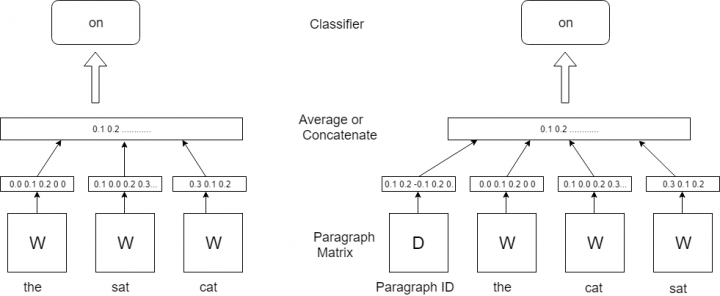Word Embeddings Machine Learning Frameworks: word2vec and doc2vec

If you need information about word2vec here are some posts:
word2vec –
Vector Representation of Text – Word Embeddings with word2vec
word2vec application –
Text Analytics Techniques with Embeddings
Using Pretrained Word Embeddinigs in Machine Learning
K Means Clustering Example with Word2Vec in Data Mining or Machine Learning

The vectors generated by doc2vec can be used for tasks like finding similarity between sentences / paragraphs / documents.  With doc2vec you can get vector for sentence or paragraph out of model without additional computations as you would do it in word2vec, for example here we used function to go from word level to sentence level:
Text Clustering with Word Embedding in Machine Learning

word2vec was very successful and it created idea to convert many other specific texts to vector. It can called “anything to vector”. So there are many different word embedding models that like doc2vec can convert more than one word to numeric vector.  Here are few examples:

tweet2vec Tweet2Vec: Character-Based Distributed Representations for Social Media
lda2vec Mixing Dirichlet Topic Models and Word Embeddings to Make lda2vec. Here is proposed model that learns dense word vectors jointly with Dirichlet-distributed latent document-level mixtures of topic vectors.
Topic2Vec Learning Distributed Representations of Topics
Med2vec Multi-layer Representation Learning for Medical Concepts
The list can go on. In the next section we will look how to load doc2vec and use for text clustering.

## Building doc2vec Model

Here is the example for converting word paragraph to vector using own built doc2vec model. The example is taken from .

The script consists of the following main steps:

• build model using own text
• save model to file
• load model from this file
• infer vector representation
```
from gensim.test.utils import common_texts
from gensim.models.doc2vec import Doc2Vec, TaggedDocument

print (common_texts)

"""
output:
[['human', 'interface', 'computer'], ['survey', 'user', 'computer', 'system', 'response', 'time'], ['eps', 'user', 'interface', 'system'], ['system', 'human', 'system', 'eps'], ['user', 'response', 'time'], ['trees'], ['graph', 'trees'], ['graph', 'minors', 'trees'], ['graph', 'minors', 'survey']]
"""

documents = [TaggedDocument(doc, [i]) for i, doc in enumerate(common_texts)]

print (documents)
"""
output
[TaggedDocument(words=['human', 'interface', 'computer'], tags=), TaggedDocument(words=['survey', 'user', 'computer', 'system', 'response', 'time'], tags=), TaggedDocument(words=['eps', 'user', 'interface', 'system'], tags=), TaggedDocument(words=['system', 'human', 'system', 'eps'], tags=), TaggedDocument(words=['user', 'response', 'time'], tags=), TaggedDocument(words=['trees'], tags=), TaggedDocument(words=['graph', 'trees'], tags=), TaggedDocument(words=['graph', 'minors', 'trees'], tags=), TaggedDocument(words=['graph', 'minors', 'survey'], tags=)]

"""

model = Doc2Vec(documents, size=5, window=2, min_count=1, workers=4)
#Persist a model to disk:

from gensim.test.utils import get_tmpfile
fname = get_tmpfile("my_doc2vec_model")

print (fname)
#output: C:\Users\userABC\AppData\Local\Temp\my_doc2vec_model

#load model from saved file
model.save(fname)
model = Doc2Vec.load(fname)
# you can continue training with the loaded model!
#If you’re finished training a model (=no more updates, only querying, reduce memory usage), you can do:

model.delete_temporary_training_data(keep_doctags_vectors=True, keep_inference=True)

#Infer vector for a new document:
#Here our text paragraph just 2 words
vector = model.infer_vector(["system", "response"])
print (vector)

"""
output

[-0.08390492  0.01629403 -0.08274432  0.06739668 -0.07021132]

"""
```

## Using Pretrained doc2vec Model

We can skip building embedding file step and use already built file. Here is an example how to do coding with pretrained word embedding file for representing test docs as vectors. The script is based on .

The below script is using pretrained on Wikipedia data doc2vec model from this location

Here is the link where you can find links to different pre-trained doc2vec and word2vec models and additional information.

You need to download zip file, unzip , put 3 files at some folder and provide path in the script. In this example it is “doc2vec/doc2vec.bin”

The main steps of the below script consist of just load doc2vec model and infer vectors.

```
import gensim.models as g
import codecs

model="doc2vec/doc2vec.bin"
test_docs="data/test_docs.txt"
output_file="data/test_vectors.txt"

#inference hyper-parameters
start_alpha=0.01
infer_epoch=1000

#load model
m = g.Doc2Vec.load(model)
test_docs = [ x.strip().split() for x in codecs.open(test_docs, "r", "utf-8").readlines() ]

#infer test vectors
output = open(output_file, "w")
for d in test_docs:
output.write( " ".join([str(x) for x in m.infer_vector(d, alpha=start_alpha, steps=infer_epoch)]) + "\n" )
output.flush()
output.close()

"""
output file
0.03772797 0.07995503 -0.1598981 0.04817521 0.033129826 -0.06923918 0.12705861 -0.06330753 .........
"""
```

So we got output file with vectors (one per each paragraph). That means we successfully converted our text to vectors. Now we can use it for different machine learning algorithms such as text classification, text clustering and many other. Next section will show example for Birch clustering algorithm with word embeddings.

## Using Pretrained doc2vec Model for Text Clustering (Birch Algorithm)

In this example we use Birch clustering algorithm for clustering text data file from 
Birch is unsupervised algorithm that is used for hierarchical clustering. An advantage of this algorithm is its ability to incrementally and dynamically cluster incoming data 

We use the following steps here:

• Load doc2vec model
• Load text docs that will be clustered
• Convert docs to vectors (infer_vector)
• Do clustering
```from sklearn import metrics

import gensim.models as g
import codecs

model="doc2vec/doc2vec.bin"
test_docs="data/test_docs.txt"

#inference hyper-parameters
start_alpha=0.01
infer_epoch=1000

#load model
m = g.Doc2Vec.load(model)
test_docs = [ x.strip().split() for x in codecs.open(test_docs, "r", "utf-8").readlines() ]

print (test_docs)
"""
[['the', 'cardigan', 'welsh', 'corgi'........
"""

X=[]
for d in test_docs:

X.append( m.infer_vector(d, alpha=start_alpha, steps=infer_epoch) )

k=3

from sklearn.cluster import Birch

brc = Birch(branching_factor=50, n_clusters=k, threshold=0.1, compute_labels=True)
brc.fit(X)

clusters = brc.predict(X)

labels = brc.labels_

print ("Clusters: ")
print (clusters)

silhouette_score = metrics.silhouette_score(X, labels, metric='euclidean')

print ("Silhouette_score: ")
print (silhouette_score)

"""
Clusters:
[1 0 0 1 1 2 1 0 1 1]
Silhouette_score:
0.17644188
"""

```

If you want to get some test with text clustering and word embeddings here is the online demo Currently it is using word2vec and glove models and k means clustering algorithm. Select ‘Text Clustering’ option and scroll down to input data.

## Conclusion

We looked what is doc2vec is, we investigated 2 ways to load this model: we can create embedding model file from our text or use pretrained embedding file. We applied doc2vec to do Birch algorithm for text clustering. In case we need to work with paragraph / sentences / docs, doc2vec can simplify word embedding for converting text to vectors.

## Text Clustering with Word Embedding in Machine Learning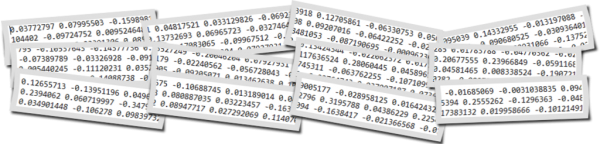Text clustering is widely used in many applications such as recommender systems, sentiment analysis, topic selection, user segmentation. Word embeddings (for example word2vec) allow to exploit ordering
of the words and semantics information from the text corpus. In this blog you can find several posts dedicated different word embedding models:

In contrast to last post from the above list, in this post we will discover how to do text clustering with word embeddings at sentence (phrase) level. The sentence could be a few words, phrase or paragraph like tweet. For examples we have 1000 of tweets and want to group in several clusters. So each cluster would contain one or more tweets.

## Data

Our data will be the set of sentences (phrases) containing 2 topics as below:
Note: I highlighted in bold 3 sentences on weather topic, all other sentences have totally different topic.
sentences = [[‘this’, ‘is’, ‘the’, ‘one’,’good’, ‘machine’, ‘learning’, ‘book’],
[‘this’, ‘is’, ‘another’, ‘book’],
[‘one’, ‘more’, ‘book’],
[‘weather’, ‘rain’, ‘snow’],
[‘yesterday’, ‘weather’, ‘snow’],
[‘forecast’, ‘tomorrow’, ‘rain’, ‘snow’],

[‘this’, ‘is’, ‘the’, ‘new’, ‘post’],
[‘this’, ‘is’, ‘about’, ‘more’, ‘machine’, ‘learning’, ‘post’],
[‘and’, ‘this’, ‘is’, ‘the’, ‘one’, ‘last’, ‘post’, ‘book’]]

## Word Embedding Method

For embeddings we will use gensim word2vec model. There is also doc2vec model – but we will use it at next post.
With the need to do text clustering at sentence level there will be one extra step for moving from word level to sentence level. For each sentence from the set of sentences, word embedding of each word is summed and in the end divided by number of words in the sentence. So we are getting average of all word embeddings for each sentence and use them as we would use embeddings at word level – feeding to machine learning clustering algorithm such k-means.

Here is the example of the function that doing this:

```def sent_vectorizer(sent, model):
sent_vec =[]
numw = 0
for w in sent:
try:
if numw == 0:
sent_vec = model[w]
else:
sent_vec = np.add(sent_vec, model[w])
numw+=1
except:
pass

return np.asarray(sent_vec) / numw
```

Now we will use text clustering Kmeans algorithm with word2vec model for embeddings. For kmeans algorithm we will use 2 separate implementations with different libraries NLTK for KMeansClusterer and sklearn for cluster. This was described in previous posts (see the list above).

The code for this article can be found in the end of this post. We use 2 for number of clusters in both k means text clustering algorithms.
Additionally we will plot data using tSNE.

## Output

Below are results

[1, 1, 1, 0, 0, 0, 1, 1, 1]

Cluster id and sentence:
1:[‘this’, ‘is’, ‘the’, ‘one’, ‘good’, ‘machine’, ‘learning’, ‘book’]
1:[‘this’, ‘is’, ‘another’, ‘book’]
1:[‘one’, ‘more’, ‘book’]
0:[‘weather’, ‘rain’, ‘snow’]
0:[‘yesterday’, ‘weather’, ‘snow’]
0:[‘forecast’, ‘tomorrow’, ‘rain’, ‘snow’]

1:[‘this’, ‘is’, ‘the’, ‘new’, ‘post’]
1:[‘this’, ‘is’, ‘about’, ‘more’, ‘machine’, ‘learning’, ‘post’]
1:[‘and’, ‘this’, ‘is’, ‘the’, ‘one’, ‘last’, ‘post’, ‘book’]

Score (Opposite of the value of X on the K-means objective which is Sum of distances of samples to their closest cluster center):
-0.0008175040203510163
Silhouette_score:
0.3498247

Cluster id and sentence:
1 [‘this’, ‘is’, ‘the’, ‘one’, ‘good’, ‘machine’, ‘learning’, ‘book’]
1 [‘this’, ‘is’, ‘another’, ‘book’]
1 [‘one’, ‘more’, ‘book’]
0 [‘weather’, ‘rain’, ‘snow’]
0 [‘yesterday’, ‘weather’, ‘snow’]
0 [‘forecast’, ‘tomorrow’, ‘rain’, ‘snow’]

1 [‘this’, ‘is’, ‘the’, ‘new’, ‘post’]
1 [‘this’, ‘is’, ‘about’, ‘more’, ‘machine’, ‘learning’, ‘post’]
1 [‘and’, ‘this’, ‘is’, ‘the’, ‘one’, ‘last’, ‘post’, ‘book’]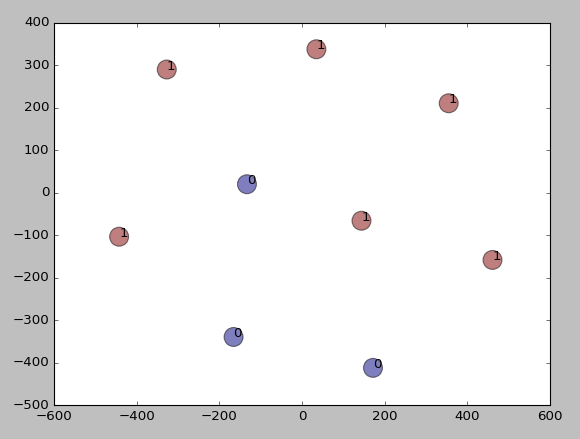Results of text clustering

We see that the data were clustered according to our expectation – different sentences by topic appeared to different clusters. Thus we learned how to do clustering algorithms in data mining or machine learning with word embeddings at sentence level. Here we used kmeans clustering and word2vec embedding model. We created additional function to go from word embeddings to sentence embeddings level. In the next post we will use doc2vec and will not need this function.

Below is full source code python script.

```from gensim.models import Word2Vec

from nltk.cluster import KMeansClusterer
import nltk
import numpy as np

from sklearn import cluster
from sklearn import metrics

# training data

sentences = [['this', 'is', 'the', 'one','good', 'machine', 'learning', 'book'],
['this', 'is',  'another', 'book'],
['one', 'more', 'book'],
['weather', 'rain', 'snow'],
['yesterday', 'weather', 'snow'],
['forecast', 'tomorrow', 'rain', 'snow'],
['this', 'is', 'the', 'new', 'post'],
['this', 'is', 'about', 'more', 'machine', 'learning', 'post'],
['and', 'this', 'is', 'the', 'one', 'last', 'post', 'book']]

model = Word2Vec(sentences, min_count=1)

def sent_vectorizer(sent, model):
sent_vec =[]
numw = 0
for w in sent:
try:
if numw == 0:
sent_vec = model[w]
else:
sent_vec = np.add(sent_vec, model[w])
numw+=1
except:
pass

return np.asarray(sent_vec) / numw

X=[]
for sentence in sentences:
X.append(sent_vectorizer(sentence, model))

print ("========================")
print (X)

# note with some version you would need use this (without wv)
#  model[model.vocab]
print (model[model.wv.vocab])

print (model.similarity('post', 'book'))
print (model.most_similar(positive=['machine'], negative=[], topn=2))

NUM_CLUSTERS=2
kclusterer = KMeansClusterer(NUM_CLUSTERS, distance=nltk.cluster.util.cosine_distance, repeats=25)
assigned_clusters = kclusterer.cluster(X, assign_clusters=True)
print (assigned_clusters)

for index, sentence in enumerate(sentences):
print (str(assigned_clusters[index]) + ":" + str(sentence))

kmeans = cluster.KMeans(n_clusters=NUM_CLUSTERS)
kmeans.fit(X)

labels = kmeans.labels_
centroids = kmeans.cluster_centers_

print ("Cluster id labels for inputted data")
print (labels)
print ("Centroids data")
print (centroids)

print ("Score (Opposite of the value of X on the K-means objective which is Sum of distances of samples to their closest cluster center):")
print (kmeans.score(X))

silhouette_score = metrics.silhouette_score(X, labels, metric='euclidean')

print ("Silhouette_score: ")
print (silhouette_score)

import matplotlib.pyplot as plt

from sklearn.manifold import TSNE

model = TSNE(n_components=2, random_state=0)
np.set_printoptions(suppress=True)

Y=model.fit_transform(X)

plt.scatter(Y[:, 0], Y[:, 1], c=assigned_clusters, s=290,alpha=.5)

for j in range(len(sentences)):
plt.annotate(assigned_clusters[j],xy=(Y[j], Y[j]),xytext=(0,0),textcoords='offset points')
print ("%s %s" % (assigned_clusters[j],  sentences[j]))

plt.show()
```

## Document Similarity, Tokenization and Word Vectors in Python with spaCY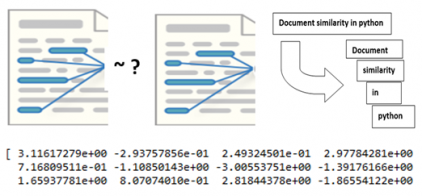Calculating document similarity is very frequent task in Information Retrieval or Text Mining. Years ago we would need to build a document-term matrix or term-document matrix that describes the frequency of terms that occur in a collection of documents and then do word vectors math to find similarity. Now by using spaCY it can be done just within few lines. Below you will find how to get document similarity , tokenization and word vectors with spaCY.

spaCY is an open-source library designed to help you build NLP applications. It has a lot of features, we will look in this post only at few but very useful.

## Document Similarity

Here is how to get document similarity:

```import spacy
nlp = spacy.load('en')

doc1 = nlp(u'Hello this is document similarity calculation')
doc2 = nlp(u'Hello this is python similarity calculation')
doc3 = nlp(u'Hi there')

print (doc1.similarity(doc2))
print (doc2.similarity(doc3))
print (doc1.similarity(doc3))

Output:
0.94
0.33
0.30
```

In more realistic situations we would load documents from files and would have longer text. Here is the experiment that I performed. I saved 3 articles from different random sites, two about deep learning and one about feature engineering.

```def get_file_contents(filename):
with open(filename, 'r') as filehandle:
filecontent = filehandle.read()
return (filecontent)

fn1="deep_learning1.txt"
fn2="feature_eng.txt"
fn3="deep_learning.txt"

fn1_doc=get_file_contents(fn1)
print (fn1_doc)

fn2_doc=get_file_contents(fn2)
print (fn2_doc)

fn3_doc=get_file_contents(fn3)
print (fn3_doc)

doc1 = nlp(fn1_doc)
doc2 = nlp(fn2_doc)
doc3 = nlp(fn3_doc)

print ("dl1 - features")
print (doc1.similarity(doc2))
print ("feature - dl")
print (doc2.similarity(doc3))
print ("dl1 - dl")
print (doc1.similarity(doc3))

"""
output:
dl1 - features
0.9700237040142454
feature - dl
0.9656364096761337
dl1 - dl
0.9547075478662724
"""

```

It was able to assign higher similarity score for documents with similar topics!

## Tokenization

Another very useful and simple feature that can be done with spaCY is tokenization. Here is how easy to convert text into tokens (words):

```for token in doc1:
print(token.text)
print (token.vector)
```

## Word Vectors

spaCY has integrated word vectors support, while other libraries like NLTK do not have it. Below line will print word embeddings – array of 768 numbers on my environment.

```
print (token.vector)   #-  prints word vector form of token.
print (doc1.vector) #- prints word vector form of first token of document.
print (doc1.vector)    #- prints mean vector form for doc1
```

So we looked how to use few features (similarity, tokenization and word embeddings) which are very easy to implement with spaCY. I hope you enjoyed this post. If you have any tips or anything else to add, please leave a comment below.

References
1. spaCY
2. Word Embeddings in Python with Spacy and Gensim

## FastText Word Embeddings for Text Classification with MLP and Python

Word embeddings are widely used now in many text applications or natural language processing moddels. In the previous posts I showed examples how to use word embeddings from word2vec Google, glove models for different tasks including machine learning clustering:

GloVe – How to Convert Word to Vector with GloVe and Python

word2vec application – K Means Clustering Example with Word2Vec in Data Mining or Machine Learning

In this post we will look at fastText word embeddings in machine learning. You will learn how to load pretrained fastText, get text embeddings and do text classification. As stated on fastText site – text classification is a core problem to many applications, like spam detection, sentiment analysis or smart replies. 

### What is fastText

fastText is an open-source, free, lightweight library that allows users to learn text representations and text classifiers. 

fastText, is created by Facebook’s AI Research (FAIR) lab. The model is an unsupervised learning algorithm for obtaining vector representations for words. Facebook makes available pretrained models for 294 languages.

As per Quora , Fasttext treats each word as composed of character ngrams. So the vector for a word is made of the sum of this character n grams. Word2vec (and glove) treat words as the smallest unit to train on. This means that fastText can generate better word embeddings for rare words. Also fastText can generate word embeddings for out of vocabulary word but word2vec and glove can not do this.

### Word Embeddings File

I downloaded wiki file wiki-news-300d-1M.vec from here , but there are some other links where you can download different data files. I found this one has smaller size so it is easy to work with it.

### Basic Operations with fastText Word Embeddings

To get most similar words to some word:

```from gensim.models import KeyedVectors
model = KeyedVectors.load_word2vec_format('wiki-news-300d-1M.vec')
print (model.most_similar('desk'))

"""
[('desks', 0.7923153638839722), ('Desk', 0.6869951486587524), ('desk.', 0.6602819561958313), ('desk-', 0.6187258958816528), ('credenza', 0.5955315828323364), ('roll-top', 0.5875717401504517), ('rolltop', 0.5837830305099487), ('bookshelf', 0.5758029222488403), ('Desks', 0.5755287408828735), ('sofa', 0.5617446899414062)]
"""
```

Load words in vocabulary:

```words = []
for word in model.vocab:
words.append(word)
```

To see embeddings:

```print("Vector components of a word: {}".format(
model[words]
))

"""
Vector components of a word: [-0.0451  0.0052  0.0776 -0.028   0.0289  0.0449  0.0117 -0.0333  0.1055
.......................................
-0.1368 -0.0058 -0.0713]
"""
```

### The Problem

So here we will use fastText word embeddings for text classification of sentences. For this classification we will use sklean Multi-layer Perceptron classifier (MLP).
The sentences are prepared and inserted into script:

```sentences = [['this', 'is', 'the', 'good', 'machine', 'learning', 'book'],
['this', 'is',  'another', 'machine', 'learning', 'book'],
['one', 'more', 'new', 'book'],

['this', 'is', 'about', 'machine', 'learning', 'post'],
['orange', 'juice', 'is', 'the', 'liquid', 'extract', 'of', 'fruit'],
['orange', 'juice', 'comes', 'in', 'several', 'different', 'varieties'],
['this', 'is', 'the', 'last', 'machine', 'learning', 'book'],
['orange', 'juice', 'comes', 'in', 'several', 'different', 'packages'],
['orange', 'juice', 'is', 'liquid', 'extract', 'from', 'fruit', 'on', 'orange', 'tree']]
```

The sentences belong to two classes, the labels for classes will be assigned later as 0,1. So our problem is to classify above sentences. Below is the flowchart of the program that we will use for perceptron learning algorithm example.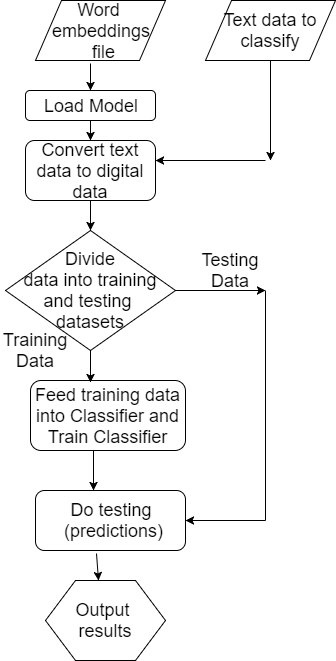Text classification using word embeddings

### Data Preparation

I converted this text input into digital using the following code. Basically I got word embedidings and averaged all words in the sentences. The resulting vector sentence representations were saved to array V.

```import numpy as np

def sent_vectorizer(sent, model):
sent_vec =[]
numw = 0
for w in sent:
try:
if numw == 0:
sent_vec = model[w]
else:
sent_vec = np.add(sent_vec, model[w])
numw+=1
except:
pass

return np.asarray(sent_vec) / numw

V=[]
for sentence in sentences:
V.append(sent_vectorizer(sentence, model))
```

After converting text into vectors we can divide data into training and testing datasets and attach class labels.

```X_train = V[0:6]
X_test = V[6:9]

Y_train = [0, 0, 0, 0, 1,1]
Y_test =  [0,1,1]
```

### Text Classification

Now it is time to load data to MLP Classifier to do text classification.

```from sklearn.neural_network import MLPClassifier
classifier = MLPClassifier(alpha = 0.7, max_iter=400)
classifier.fit(X_train, Y_train)

df_results = pd.DataFrame(data=np.zeros(shape=(1,3)), columns = ['classifier', 'train_score', 'test_score'] )
train_score = classifier.score(X_train, Y_train)
test_score = classifier.score(X_test, Y_test)

print  (classifier.predict_proba(X_test))
print  (classifier.predict(X_test))

df_results.loc[1,'classifier'] = "MLP"
df_results.loc[1,'train_score'] = train_score
df_results.loc[1,'test_score'] = test_score

print(df_results)

"""
Output
classifier  train_score  test_score
MLP          1.0         1.0
"""
```

In this post we learned how to use pretrained fastText word embeddings for converting text data into vector model. We also looked how to load word embeddings into machine learning algorithm. And in the end of post we looked at machine learning text classification using MLP Classifier with our fastText word embeddings. You can find full python source code and references below.

```from gensim.models import KeyedVectors
import pandas as pd

model = KeyedVectors.load_word2vec_format('wiki-news-300d-1M.vec')
print (model.most_similar('desk'))

words = []
for word in model.vocab:
words.append(word)

print("Vector components of a word: {}".format(
model[words]
))
sentences = [['this', 'is', 'the', 'good', 'machine', 'learning', 'book'],
['this', 'is',  'another', 'machine', 'learning', 'book'],
['one', 'more', 'new', 'book'],
['this', 'is', 'about', 'machine', 'learning', 'post'],
['orange', 'juice', 'is', 'the', 'liquid', 'extract', 'of', 'fruit'],
['orange', 'juice', 'comes', 'in', 'several', 'different', 'varieties'],
['this', 'is', 'the', 'last', 'machine', 'learning', 'book'],
['orange', 'juice', 'comes', 'in', 'several', 'different', 'packages'],
['orange', 'juice', 'is', 'liquid', 'extract', 'from', 'fruit', 'on', 'orange', 'tree']]

import numpy as np

def sent_vectorizer(sent, model):
sent_vec =[]
numw = 0
for w in sent:
try:
if numw == 0:
sent_vec = model[w]
else:
sent_vec = np.add(sent_vec, model[w])
numw+=1
except:
pass

return np.asarray(sent_vec) / numw

V=[]
for sentence in sentences:
V.append(sent_vectorizer(sentence, model))

X_train = V[0:6]
X_test = V[6:9]
Y_train = [0, 0, 0, 0, 1,1]
Y_test =  [0,1,1]

from sklearn.neural_network import MLPClassifier
classifier = MLPClassifier(alpha = 0.7, max_iter=400)
classifier.fit(X_train, Y_train)

df_results = pd.DataFrame(data=np.zeros(shape=(1,3)), columns = ['classifier', 'train_score', 'test_score'] )
train_score = classifier.score(X_train, Y_train)
test_score = classifier.score(X_test, Y_test)

print  (classifier.predict_proba(X_test))
print  (classifier.predict(X_test))

df_results.loc[1,'classifier'] = "MLP"
df_results.loc[1,'train_score'] = train_score
df_results.loc[1,'test_score'] = test_score
print(df_results)
```

## How to Convert Word to Vector with GloVe and Python

In the previous post we looked at Vector Representation of Text with word embeddings using word2vec. Another approach that can be used to convert word to vector is to use GloVe – Global Vectors for Word Representation. Per documentation from home page of GloVe  “GloVe is an unsupervised learning algorithm for obtaining vector representations for words. Training is performed on aggregated global word-word co-occurrence statistics from a corpus”. Thus we can convert word to vector using GloVe.

At this post we will look how to use pretrained GloVe data file that can be downloaded from .We will look how to get word vector representation from this downloaded datafile. We will also look how to get nearest words. Why do we need vector representation of text? Because this is what we input to machine learning or data science algorithms – we feed numerical vectors to algorithms such as text classification, machine learning clustering or other text analytics algorithms.

### Loading Glove Datafile

The code that I put here is based on some examples that I found on StackOverflow .

So first you need to open the file and load data into the model. Then you can get the vector representation and other things.

Below is the full source code for glove python script:

```file = "C:\\Users\\glove\\glove.6B.50d.txt"
import numpy as np
def loadGloveModel(gloveFile):
print ("Loading Glove Model")

with open(gloveFile, encoding="utf8" ) as f:
content = f.readlines()
model = {}
for line in content:
splitLine = line.split()
word = splitLine
embedding = np.array([float(val) for val in splitLine[1:]])
model[word] = embedding
print ("Done.",len(model)," words loaded!")
return model

model= loadGloveModel(file)

print (model['hello'])

"""
Below is the output of the above code
Loading Glove Model
Done. 400000  words loaded!
[-0.38497   0.80092   0.064106 -0.28355  -0.026759 -0.34532  -0.64253
-0.11729  -0.33257   0.55243  -0.087813  0.9035    0.47102   0.56657
0.6985   -0.35229  -0.86542   0.90573   0.03576  -0.071705 -0.12327
0.54923   0.47005   0.35572   1.2611   -0.67581  -0.94983   0.68666
0.3871   -1.3492    0.63512   0.46416  -0.48814   0.83827  -0.9246
-0.33722   0.53741  -1.0616   -0.081403 -0.67111   0.30923  -0.3923
-0.55002  -0.68827   0.58049  -0.11626   0.013139 -0.57654   0.048833
0.67204 ]
"""
```

So we got numerical representation of word ‘hello’.
We can use also pandas to load GloVe file. Below are functions for loading with pandas and getting vector information.

```import pandas as pd
import csv

words = pd.read_table(file, sep=" ", index_col=0, header=None, quoting=csv.QUOTE_NONE)

def vec(w):
return words.loc[w].as_matrix()

print (vec('hello'))    #this will print same as print (model['hello'])  before

```

### Finding Closest Word or Words

Now how do we find closest word to word “table”? We iterate through pandas dataframe, find deltas and then use numpy argmin function.
The closest word to some word will be always this word itself (as delta = 0) so I needed to drop the word ‘table’ and also next closest word ‘tables’. The final output for the closest word was “place”

```words = words.drop("table", axis=0)
words = words.drop("tables", axis=0)

words_matrix = words.as_matrix()

def find_closest_word(v):
diff = words_matrix - v
delta = np.sum(diff * diff, axis=1)
i = np.argmin(delta)
return words.iloc[i].name

print (find_closest_word(model['table']))
#output:  place

#If we want retrieve more than one closest words here is the function:

def find_N_closest_word(v, N, words):
Nwords=[]
for w in range(N):
diff = words.as_matrix() - v
delta = np.sum(diff * diff, axis=1)
i = np.argmin(delta)
Nwords.append(words.iloc[i].name)
words = words.drop(words.iloc[i].name, axis=0)

return Nwords

print (find_N_closest_word(model['table'], 10, words))

#Output:
#['table', 'tables', 'place', 'sit', 'set', 'hold', 'setting', 'here', 'placing', 'bottom']
```

We can also use gensim word2vec library functionalities after we load GloVe file.

```from gensim.scripts.glove2word2vec import glove2word2vec
glove2word2vec(glove_input_file=file, word2vec_output_file="gensim_glove_vectors.txt")

###Finally, read the word2vec txt to a gensim model using KeyedVectors:

from gensim.models.keyedvectors import KeyedVectors
glove_model = KeyedVectors.load_word2vec_format("gensim_glove_vectors.txt", binary=False)

```

### Difference between word2vec and GloVe

Both models learn geometrical encodings (vectors) of words from their co-occurrence information. They differ in the way how they learn this information. word2vec is using a “predictive” model (feed-forward neural network), whereas GloVe is using a “count-based” model (dimensionality reduction on the co-occurrence counts matrix). 

I hope you enjoyed reading this post about how to convert word to vector with GloVe and python. If you have any tips or anything else to add, please leave a comment below.